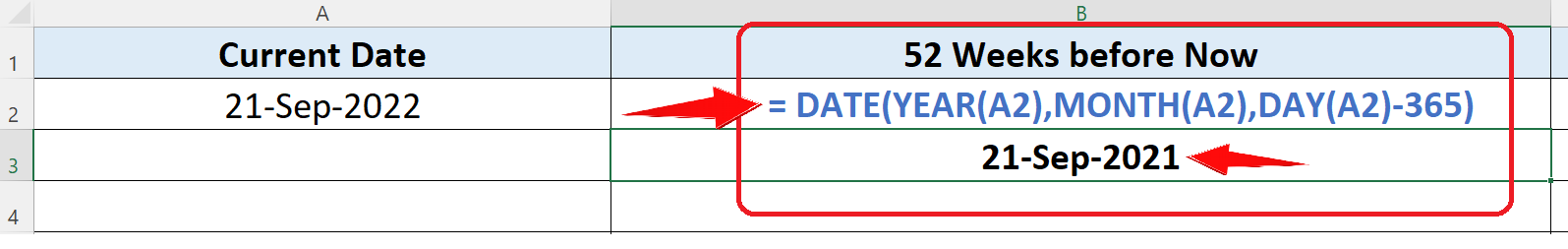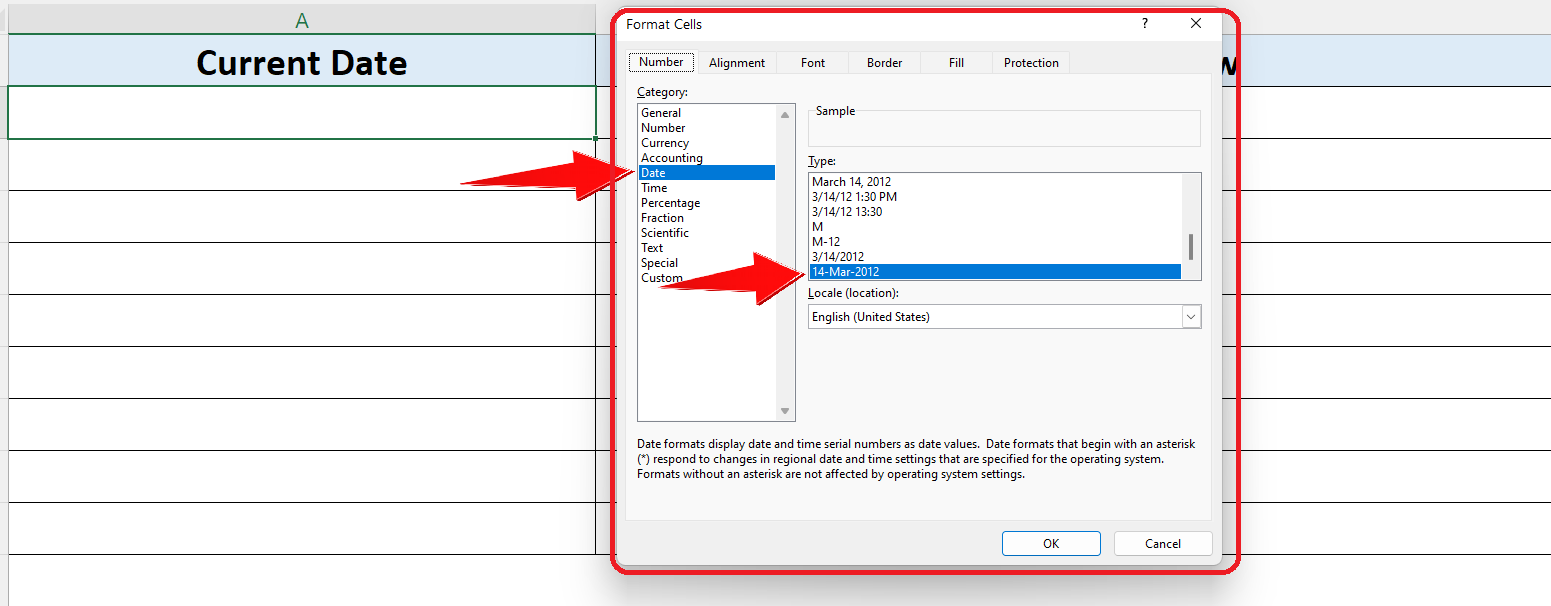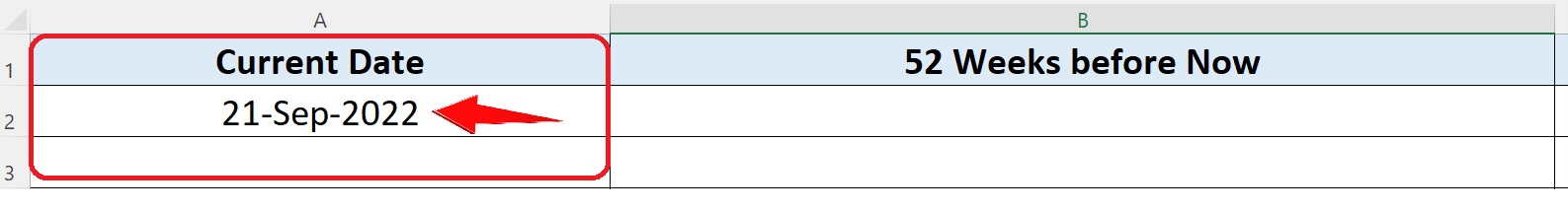# How to calculate the date of 52 weeks ago (one Year) from now in ExcelWhile we are working with dates in excel, often we have to add or subtract the dates from each other. Sometimes we want to calculate a specific date in the past with reference to a date in our data set. So let’s look at a specific example in which we’ll find a date which is 52 weeks in the past (i.e. one year ago) from today. Let’s see how to do this step by step;

### Step 1 – Choose a friendly date format– First we’ll choose a friendly date format to understand the date easily. To do this select a cell in which you wish to display the current date and press CTRL+1.

– This will open up the format cell menu and use this formula as shown above.

### Step 2 – Find the current date (today’s date)– Let’s find the current date. To do this select a cell in which you wish to display the current date and implement this formula shown above;

= TODAY()

– This will show the current date in the cell where we wrote the formula as shown in the figure above.

### Step 3 – Find the date 52 weeks before now (one year before)– To find the date which is 13 weeks from now we’ll have to use Excel’s built-in DATE formula. To do this select a cell in which you wish to find the future date (13 weeks) and implement this formula shown below;

=DATE(YEAR(A2),MONTH(A2),DAY(A2)-365)

Let’s implement this formula as shown in below picture;

## Break down of the formula:

We used the DATE function of Excel and the format of the DATE function is as follows;

DATE (year, month, day)

This function is used to create a date using a given year, month and day. When we passed on A2 (holding today’s date) as a parameter to this function in each argument then it used the year ofA2 (2022), month of A2 (Sep) and day of A2 (today) as well to create a date. However, we wished to find out a date which is 52 weeks before today, which is exactly one year before today. So we subtracted 365 days in the day section of the formula because 52 weeks are equal to 365 days. So this is how we found out a date that is 52 weeks in the past from today. Using this technique we can find any date in the past with reference to any specific date.